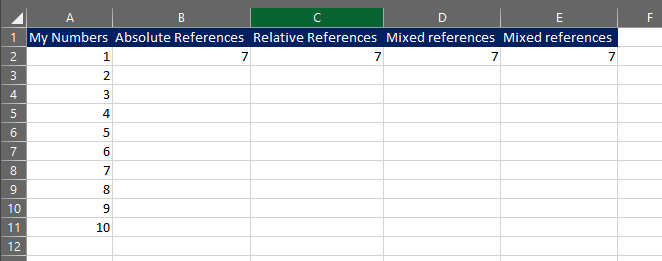# How to write excel formulas from VBA? //Formula, FormulaR1C1//

This section will go through the different options when it comes to writing out the formula

instead of just the values with VBA.VBA typically writes out the values, however sometimes you might want to show the calculation that is being made in VBA for the end user. It is slightly more time consuming than simply writing out the values but not too difficult. There 2 main concepts for this.

1. Sheet formula - just as you are used to. The only difference in VBA is that you have to write it out as text since the formula starts with '=' sign.

2. FormulaR1C1 style formula - this is a slightly different concept compared to the normal sheet formula method. However, this is very practical for relative cell references, which has mainly been my use for this method. R1C1 stands for Rows and Columns.

### Cell references ​

On top of the different ways to write the formulas in VBA, you might also consider which cell reference you want to use, which can be either absolute (\$), relative, or a mixed version. In the below code I am picking cell A8 in excel with the different absolute/relative references option in the R1C1 style. In many cases writing formulas to excel you might need to include the .Formula or .FormulaR1C1 syntax at the end of your range to specify which formula. I didn't need to now, but illustrate this in my first example below. In older excel versions this is most likely needed.

```Sub Formula_RC_Style()

'Brackets in the R1C1 formula style is the factor that denotes the difference between using a relative reference vs using an absolute reference. Without the brackets [ ], you are creating a relative formula.

'Cell A8 - absolute reference
'=\$A\$8
Range("B2").Formula = "=R8C1"

'Cell A8 - relative reference from cell A1
'=\$A\$8
Range("C2") = "=RC[-2]"

'Cell A8 - relative reference from cell A1 (Relative row, Absolute column)
'=\$A\$8
Range("D2") = "=RC1"

'Cell A8 - relative reference from cell A1 (Absolute row, Relative column)
'=\$A\$8
Range("E2") = "=R8C[-4]"

End Sub```

### How my worksheet looks like after I run the macro### Sum in the different formula styles

Below you will find the code for creating a sum with the different formula options. Now this would of course work with any other formula as well. Such as Vlookup, Sumif, Countif etc.. I just happened to choose sum.

```Sub Formula_Sum()

'Formula by text.
'Works with any excel formula as it is just text printed out since it is using "" markers.
Range("B2") = "=Sum(A2:A11)"
​
'Formula with R1C1 notation - relative reference (in this case relative to cell C2.
'Using R1C1 style notation relative to position instead.
Range("C2") = "=SUM(RC[-2]:RC[-2])"

'Formula with R1C1 notation - absolute reference
Range("D2") = "=SUM(R2C1:R11C1)"

End Sub```

### How my worksheet looks like after I run the macro### Sum in the different sheets "!" and using variables in formulas

Below you will find the code for creating a sum using a variable, and referencing other worksheets. Sometimes it can be a bit tricky, but just mimic the formula in excel in VBA and you will be alright.

```Sub Formula_Sheet_Variable()
​
'My Codename for my sheet is MySheet
'My sheetname for my sheet is "Formula"
​
Dim MyVariable As Integer
MyVariable = 7

'Multiplication of Sum
Range("B2") = "=Sum(A2:A11)* " & MyVariable

'Cell reference with variable
Range("C2") = "=Sum(A" & MyVariable & ":A11)"

'Cell reference with different sheet or the same.
'Key is to use the '!' - sign just like you would in excel.
'If you are using older excel you might also need the "'" - signs in the beginning and after sheet name.
Range("D2") = "=Sum(Formula!A2:A11)"

'Sheet specification using code name instead. Better, and more reliable as it is less risk for maniuplation.
Range("E2") = "=Sum(" & MySheet.Name & "!A2:A11)"

End Sub```

### How my worksheet looks like after I run the macro